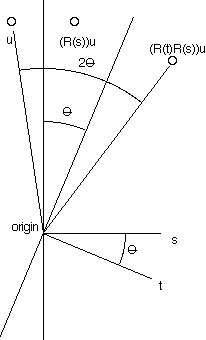One way to understand something about a collection of mathematical objects is to describe a way of generating them. We consider here rotations in n dimensions and how they can be composed of reflections.

We need a word that includes rotations and reflections. ‘Isometries’ may move the origin and is thus too inclusive. ‘Rotation’ excludes reflections, and is thus too narrow. I will coin the noun ‘ot’ (orthogonal transformation) until someone suggests a better word.

We follow ideas that Clifford perhaps first elaborated. We maintain the distinction between turns of 2π and 4π that is described here. A turn carries one bit more information than an ot. It turns out that there are just two turns for every ot, but we are getting ahead of ourselves. We take geometry as the center of ideas here but with references to the algebras of Hamilton and Clifford.

Hamilton gave us Quaternions which provide a way to compute rigid rotations in 3D not including reflections. Subsequently Clifford gave us a generalization that includes reflections and works in n dimensions. I think it is easier to include reflections.

We assume an n dimensional space, with real coordinates, that is also a vector space, over the reals, with a fixed origin.

For a fixed unit vector r the transformation of the space that takes v to v − 2(v•r)r is called a reflection which we call R(r).
(R(r))v = v − 2(v•r)r. This is the reflection of the space by the n−1 dimensional subspace that is perpendicular to r.

By an ot we mean a transformation of the space to itself that leaves the origin fixed and preserves the distance between points. A reflection is an ot. Ots form a group. Real n by n orthogonal matrices are in one-to-one correspondence with ots. Multiplication of orthogonal matrices corresponds to composing the related ots.It turns out that any ot can be composed of reflections. In 2D consider two unit vectors s and t with a small angle θ between them, and the consequences of composing their respective reflections: R(t)R(s). Observe that the composed reflections rotates a point u by angle 2θ. Observe also that the opposite composition R(s)R(t) is a rotation by −2θ.

In 2D or 3D a rotation can by had by just 2 reflections. It is not so simple in higher dimensions.

Hamilton and Clifford wanted a mathematical structure akin to the complex numbers to describe rotations. I would have been satisfied with orthogonal matrices but they had other ideas. It turns out that their intuition was right. Clifford proposed that r be taken to denote R(r) and then rs would denote R(r)R(s) but what are the multiplication rules for vectors? Hamilton’s quaternions showed the way for 3D but did not generalize. Quaternions were defined with three square roots of −1, called i, j and k.

Hamilton and Clifford’s methodology required writing these values as linear combinations for a few fixed ‘basis’ elements. The coefficients would be real. In this role 1, i, j and k sufficed for the quaternions.